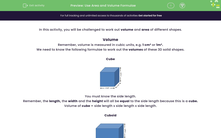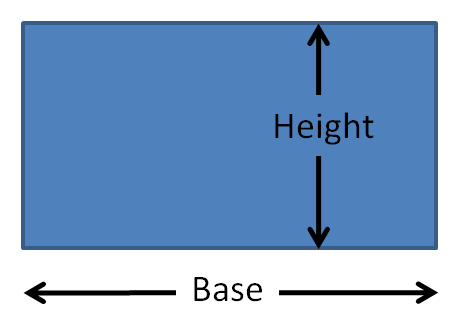# Use Area and Volume Formulae

In this worksheet, students will be asked to find the volume of cubes and cuboids and the area of rectangle and parallelograms.Key stage:  KS 2

Curriculum topic:   Measurement

Curriculum subtopic:   Use Area/Volume Formulae

Popular topics:   Area worksheets

Difficulty level:#### Worksheet Overview

In this activity, you will be challenged to work out volume and area of different shapes.

Volume

Remember, volume is measured in cubic units, e.g. 1 cm³ or 1m³.

We need to know the following formulae to work out the volumes of these 3D solid shapes.

CubeYou must know the side length.

Remember, the length, the width and the height will all be equal to the side length because this is a cube.

Volume of cube = side length x side length x side length.

CuboidYou must know:

The length

The width

The height

Volume of cuboid = length x width x height

Area

Remember area is measured in square units for example, m² or cm².

RectanglesYou must know:

The base length.

The perpendicular height from the base to the top.

Area of rectangle = base x height.

ParallelogramYou must know:

The base length

The perpendicular height from base to top (not the slant height)

Area of parallelogram = base x height

TriangleYou must know,

The base length

The perpendicular height from the base to the apex (not the slant heights)

Area of triangle = 1/2 base x height

Or

Area of triangle = base x height ÷ 2